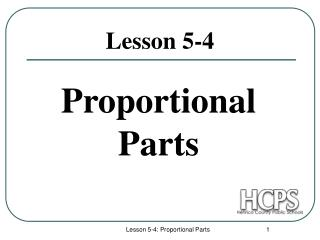# Proportional Parts - PowerPoint PPT PresentationDownload PresentationProportional Parts

Download Presentation## Proportional Parts

- - - - - - - - - - - - - - - - - - - - - - - - - - - E N D - - - - - - - - - - - - - - - - - - - - - - - - - - -
##### Presentation Transcript

1. Proportional Parts Lesson 5-4 Lesson 5-4: Proportional Parts

2. F C A B D E Two polygons are similar if and only if their corresponding angles are congruent and the measures of their corresponding sides are proportional. Similar Polygons Lesson 5-4: Proportional Parts

3. C B 1 2 D A 4 3 E Triangle Proportionality Theorem If a line is parallel to one side of a triangle and intersects the other two sides in two distinct points, then it separates these sides into segments of proportional length. Converse: If a line intersects two sides of a triangle and separates the sides into corresponding segments of proportional lengths, then the line is parallel to the third side. Lesson 5-4: Proportional Parts

4. B D E 6 9 C A x 4 B 4x + 3 2x + 3 D E 9 5 A C Examples……… Example 1: If BE = 6, EA = 4, and BD = 9, find DC. 6x = 36 x = 6 Example 2: Solve for x. Lesson 5-4: Proportional Parts

5. Theorem A segment that joins the midpoints of two sides of a triangle is parallel to the third side of the triangle, and its length is one-half the length of the third side. R M L T S Lesson 5-4: Proportional Parts

6. Corollary If three or more parallel lines have two transversals, they cut off the transversals proportionally. If three or more parallel lines cut off congruent segments on one transversal, then they cut off congruent segments on every transversal. F E D A B C Lesson 5-4: Proportional Parts

7. C A D B Theorem An angle bisector in a triangle separates the opposite side into segments that have the same ratio as the other two sides. Lesson 5-4: Proportional Parts

8. A D F E B C J H G I If two triangles are similar: (1) then the perimeters are proportional to the measures of the corresponding sides. (2) then the measures of the corresponding altitudes are proportional to the measure of the corresponding sides.. (3) then the measures of the corresponding angle bisectors of the triangles are proportional to the measures of the corresponding sides.. Lesson 5-4: Proportional Parts

9. E B F D C A 25 15 4 20 Example: Given: ΔABC ~ ΔDEF, AB = 15, AC = 20, BC = 25, and DF = 4. Find the perimeter of ΔDEF. The perimeter of ΔABC is 15 + 20 + 25 = 60. Side DF corresponds to side AC, so we can set up a proportion as: Lesson 5-4: Proportional Parts